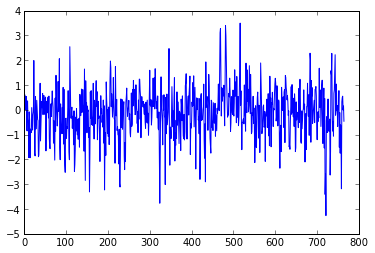# 03 NumPy arrays

This is part of Python for Geosciences notes.

================• a powerful N-dimensional array object
• tools for integrating C/C++ and Fortran code
• useful linear algebra, Fourier transform, and random number capabilities
In :
set_printoptions(precision=3 , suppress= True) # this is just to make the output look better


I am going to use some real data as an example of array manipulations. This will be the AO index downloaded by wget through a system call (you have to be on Linux of course):

In [ ]:
!wget www.cpc.ncep.noaa.gov/products/precip/CWlink/daily_ao_index/monthly.ao.index.b50.current.ascii


This is how data in the file look like (we again use system call for head command):

In :
!head monthly.ao.index.b50.current.ascii

 1950    1  -0.60310E-01
1950    2   0.62681E+00
1950    3  -0.81275E-02
1950    4   0.55510E+00
1950    5   0.71577E-01
1950    6   0.53857E+00
1950    7  -0.80248E+00
1950    8  -0.85101E+00
1950    9   0.35797E+00
1950   10  -0.37890E+00


Load data in to a variable:

In :
ao = loadtxt('monthly.ao.index.b50.current.ascii')

In :
ao

Out:
array([[ 1950.   ,     1.   ,    -0.06 ],
[ 1950.   ,     2.   ,     0.627],
[ 1950.   ,     3.   ,    -0.008],
...,
[ 2013.   ,     7.   ,    -0.011],
[ 2013.   ,     8.   ,     0.154],
[ 2013.   ,     9.   ,    -0.461]])
In :
ao.shape

Out:
(765, 3)

So it's a row-major order. Matlab and Fortran use column-major order for arrays.

In :
type(ao)

Out:
numpy.ndarray

Numpy arrays are statically typed, which allow faster operations

In :
ao.dtype

Out:
dtype('float64')

You can't assign value of different type to element of the numpy array:

In :
ao[0,0] = 'Year'

---------------------------------------------------------------------------
ValueError                                Traceback (most recent call last)
in ()
----> 1 ao[0,0] = 'Year'

ValueError: could not convert string to float: Year

Slicing works similarly to Matlab:

In :
ao[0:5,:]

Out:
array([[ 1950.   ,     1.   ,    -0.06 ],
[ 1950.   ,     2.   ,     0.627],
[ 1950.   ,     3.   ,    -0.008],
[ 1950.   ,     4.   ,     0.555],
[ 1950.   ,     5.   ,     0.072]])

One can look at the data. This is done by matplotlib module and you have to start IPython with --pylab inline option to make it work:

In :
plot(ao[:,2])

Out:
[]## Index slicing¶

In general it is similar to Matlab

First 12 elements of second column (months). Remember that indexing starts with 0:

In :
ao[0:12,1]

Out:
array([  1.,   2.,   3.,   4.,   5.,   6.,   7.,   8.,   9.,  10.,  11.,
12.])

First raw:

In :
ao[0,:]

Out:
array([ 1950.  ,     1.  ,    -0.06])

We can create mask, selecting all raws where values in second raw (months) equals 10 (October):

In :
mask = (ao[:,1]==10)


Here we apply this mask and show only first 5 rowd of the array:

In :
ao[mask][:5,:]

Out:
array([[ 1950.   ,    10.   ,    -0.379],
[ 1951.   ,    10.   ,    -0.213],
[ 1952.   ,    10.   ,    -0.437],
[ 1953.   ,    10.   ,    -0.194],
[ 1954.   ,    10.   ,     0.513]])

You don't have to create separate variable for mask, but apply it directly. Here instead of first five rows I show five last rows:

In :
ao[ao[:,1]==10][-5:,:]

Out:
array([[ 2008.   ,    10.   ,     1.676],
[ 2009.   ,    10.   ,    -1.54 ],
[ 2010.   ,    10.   ,    -0.467],
[ 2011.   ,    10.   ,     0.8  ],
[ 2012.   ,    10.   ,    -1.514]])

You can combine conditions. In this case we select October-December data (only first 10 elements are shown):

In :
ao[(ao[:,1]>=10)&(ao[:,1]<=12)][0:10,:]

Out:
array([[ 1950.   ,    10.   ,    -0.379],
[ 1950.   ,    11.   ,    -0.515],
[ 1950.   ,    12.   ,    -1.928],
[ 1951.   ,    10.   ,    -0.213],
[ 1951.   ,    11.   ,    -0.069],
[ 1951.   ,    12.   ,     1.987],
[ 1952.   ,    10.   ,    -0.437],
[ 1952.   ,    11.   ,    -1.891],
[ 1952.   ,    12.   ,    -1.827],
[ 1953.   ,    10.   ,    -0.194]])

## Basic operations¶

Create example array from first 12 values of second column and perform some basic operations:

In :
months = ao[0:12,1]
months

Out:
array([  1.,   2.,   3.,   4.,   5.,   6.,   7.,   8.,   9.,  10.,  11.,
12.])
In :
months+10

Out:
array([ 11.,  12.,  13.,  14.,  15.,  16.,  17.,  18.,  19.,  20.,  21.,
22.])
In :
months*20

Out:
array([  20.,   40.,   60.,   80.,  100.,  120.,  140.,  160.,  180.,
200.,  220.,  240.])
In :
months*months

Out:
array([   1.,    4.,    9.,   16.,   25.,   36.,   49.,   64.,   81.,
100.,  121.,  144.])

## Basic statistics¶

Create ao_values that will contain onlu data values:

In :
ao_values = ao[:,2]


Simple statistics:

In :
ao_values.min()

Out:
-4.2656999999999998
In :
ao_values.max()

Out:
3.4952999999999999
In :
ao_values.mean()

Out:
-0.13462109949019607
In :
ao_values.std()

Out:
1.0054168027600721
In :
ao_values.sum()

Out:
-102.98514111

You can also use sum function:

In :
sum(ao_values)

Out:
-102.98514111

One can make operations on the subsets:

In :
mean(ao[ao[:,1]==1,2]) # January monthly mean

Out:
-0.40406150000000002

Result will be the same if we use method on our selected data:

In :
ao[ao[:,1]==1,2].mean()

Out:
-0.40406150000000002

## Saving data¶

You can save your data as a text file

In :
savetxt('ao_only_values.csv',ao[:, 2], fmt='%.4f')


In :
!head ao_only_values.csv

-0.0603
0.6268
-0.0081
0.5551
0.0716
0.5386
-0.8025
-0.8510
0.3580
-0.3789


You can also save it as binary:

In :
f=open('ao_only_values.bin', 'w')
ao[:,2].tofile(f)
f.close()